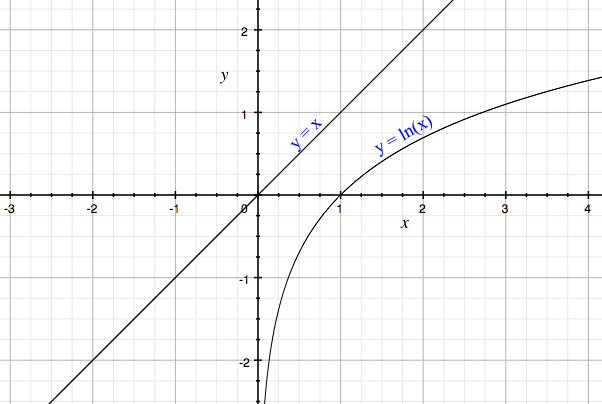SEARCH HOMEMath Central Quandaries & QueriesQuestion from Udayan, a student: Sir/Madam, How to find the solutions of the equation, x = ln(x)Hi,

If I am presented with two equations and asked to solve for the points where they intersect and I don't see an obvious algebraic solution I graph the equations to get an ida of where they intersect. For your problem I graphed $y = x$ and $y = \ln(x).$From my graph it looks like they don't intersect. Can you prove that they don't intersect?

PennyMath Central is supported by the University of Regina and The Pacific Institute for the Mathematical Sciences.